The Matrix class represents a transformation matrix that determines how to map points from one coordinate space to another. You can perform various graphical transformations on a display object by setting the properties of a Matrix object, applying that Matrix object to the `matrix` property of a Transform object, and then applying that Transform object as the `transform` property of the display object. These transformation functions include translation (x and y repositioning), rotation, scaling, and skewing. Together these types of transformations are known as affine transformations. Affine transformations preserve the straightness of lines while transforming, so that parallel lines stay parallel.

To apply a transformation matrix to a display object, you create a Transform object, set its `matrix` property to the transformation matrix, and then set the `transform` property of the display object to the Transform object. Matrix objects are also used as parameters of some methods, such as the following:

• The `draw()` method of a BitmapData object
• The `beginBitmapFill()` method, `beginGradientFill()` method, or `lineGradientStyle()` method of a Graphics object

A transformation matrix object is a 3 x 3 matrix with the following contents: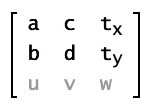In traditional transformation matrixes, the `u`, `v`, and `w` properties provide extra capabilities. The Matrix class can only operate in two-dimensional space, so it always assumes that the property values `u` and `v` are 0.0, and that the property value `w` is 1.0. The effective values of the matrix are as follows: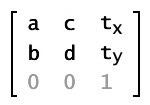You can get and set the values of all six of the other properties in a Matrix object: `a`, `b`, `c`, `d`, `tx`, and `ty`.

The Matrix class supports the four major types of transformations: translation, scaling, rotation, and skewing. You can set three of these transformations by using specialized methods, as described in the following table:

TransformationMethodMatrix valuesDisplay resultDescription
Translation (displacement)`translate(tx, ty)`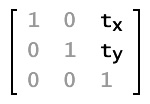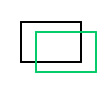Moves the image `tx` pixels to the right and `ty` pixels down.
Scaling`scale(sx, sy)`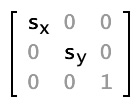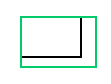Resizes the image, multiplying the location of each pixel by `sx` on the x axis and `sy` on the y axis.
Rotation`rotate(q)`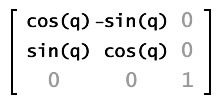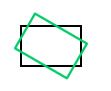Rotates the image by an angle `q`, which is measured in radians.
Skewing or shearingNone; must set the properties `b` and `c`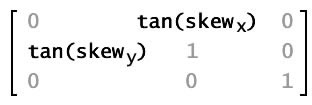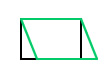Progressively slides the image in a direction parallel to the x or y axis. The `b` property of the Matrix object represents the tangent of the skew angle along the y axis; the `c` property of the Matrix object represents the tangent of the skew angle along the x axis.

Each transformation function alters the current matrix properties so that you can effectively combine multiple transformations. To do this, you call more than one transformation function before applying the matrix to its display object target (by using the `transform` property of that display object).

Use the `new Matrix()` constructor to create a Matrix object before you can call the methods of the Matrix object.

### `@:value({ ty : 0, tx : 0, d : 1, c : 0, b : 0, a : 1 })new(a:Float = 1, b:Float = 0, c:Float = 0, d:Float = 1, tx:Float = 0, ty:Float = 0)`

Creates a new Matrix object with the specified parameters. In matrix notation, the properties are organized like this:If you do not provide any parameters to the `new Matrix()` constructor, it creates an identity matrix with the following values:

`a = 1``b = 0`
`tx = 0``ty = 0`

In matrix notation, the identity matrix looks like this: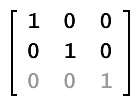Parameters:

`a` The value that affects the positioning of pixels along the x axis when scaling or rotating an image. The value that affects the positioning of pixels along the y axis when rotating or skewing an image. The value that affects the positioning of pixels along the x axis when rotating or skewing an image. The value that affects the positioning of pixels along the y axis when scaling or rotating an image.. The distance by which to translate each point along the x axis. The distance by which to translate each point along the y axis.

### `a:Float`

The value that affects the positioning of pixels along the x axis when scaling or rotating an image.

### `b:Float`

The value that affects the positioning of pixels along the y axis when rotating or skewing an image.

### `c:Float`

The value that affects the positioning of pixels along the x axis when rotating or skewing an image.

### `d:Float`

The value that affects the positioning of pixels along the y axis when scaling or rotating an image.

### `tx:Float`

The distance by which to translate each point along the x axis.

### `ty:Float`

The distance by which to translate each point along the y axis.

### `clone():Matrix`

Returns a new Matrix object that is a clone of this matrix, with an exact copy of the contained object.

Returns:

A Matrix object.

### `concat(m:Matrix):Void`

Concatenates a matrix with the current matrix, effectively combining the geometric effects of the two. In mathematical terms, concatenating two matrixes is the same as combining them using matrix multiplication.

For example, if matrix `m1` scales an object by a factor of four, and matrix `m2` rotates an object by 1.5707963267949 radians(`Math.PI/2`), then `m1.concat(m2)` transforms `m1` into a matrix that scales an object by a factor of four and rotates the object by `Math.PI/2` radians.

This method replaces the source matrix with the concatenated matrix. If you want to concatenate two matrixes without altering either of the two source matrixes, first copy the source matrix by using the `clone()` method, as shown in the Class Examples section.

Parameters:

`m` The matrix to be concatenated to the source matrix.

### `copyColumnFrom(column:Int, vector3D:Vector3D):Void`

Copies a Vector3D object into specific column of the calling Matrix3D object.

Parameters:

`column` The column from which to copy the data from. The Vector3D object from which to copy the data.

### `copyColumnTo(column:Int, vector3D:Vector3D):Void`

Copies specific column of the calling Matrix object into the Vector3D object. The `w` element of the Vector3D object will not be changed.

Parameters:

`column` The column from which to copy the data from. The Vector3D object from which to copy the data.

### `copyFrom(sourceMatrix:Matrix):Void`

Copies all of the matrix data from the source Point object into the calling Matrix object.

Parameters:

`sourceMatrix` The Matrix object from which to copy the data.

### `copyRowFrom(row:Int, vector3D:Vector3D):Void`

Copies a Vector3D object into specific row of the calling Matrix object.

Parameters:

`row` The row from which to copy the data from. The Vector3D object from which to copy the data.

### `copyRowTo(row:Int, vector3D:Vector3D):Void`

Copies specific row of the calling Matrix object into the Vector3D object. The `w` element of the Vector3D object will not be changed.

Parameters:

`row` The row from which to copy the data from. The Vector3D object from which to copy the data.

### `@:value({ ty : 0, tx : 0, rotation : 0 })createBox(scaleX:Float, scaleY:Float, rotation:Float = 0, tx:Float = 0, ty:Float = 0):Void`

Includes parameters for scaling, rotation, and translation. When applied to a matrix it sets the matrix's values based on those parameters. Using the `createBox()` method lets you obtain the same matrix as you would if you applied the `identity()`, `rotate()`, `scale()`, and `translate()` methods in succession. For example, `mat1.createBox(2,2,Math.PI/4, 100, 100)` has the same effect as the following:

``````import openfl.geom.Matrix;

var mat1 = new Matrix();
mat1.identity();
mat1.rotate(Math.PI/4);
mat1.scale(2,2);
mat1.translate(10,20);``````

Parameters:

`scaleX` The factor by which to scale horizontally. The factor by which scale vertically. The amount to rotate, in radians. The number of pixels to translate (move) to the right along the x axis. The number of pixels to translate (move) down along the y axis.

### `@:value({ ty : 0, tx : 0, rotation : 0 })createGradientBox(width:Float, height:Float, rotation:Float = 0, tx:Float = 0, ty:Float = 0):Void`

Creates the specific style of matrix expected by the `beginGradientFill()` and `lineGradientStyle()` methods of the Graphics class. Width and height are scaled to a `scaleX`/`scaleY` pair and the `tx`/`ty` values are offset by half the width and height.

For example, consider a gradient with the following characteristics:

• `GradientType.LINEAR`
• Two colors, green and blue, with the ratios array set to `[0, 255]`
• `SpreadMethod.PAD`
• `InterpolationMethod.LINEAR_RGB`

The following illustrations show gradients in which the matrix was defined using the `createGradientBox()` method with different parameter settings:

`createGradientBox()` settingsResulting gradient
`width = 25; height = 25; rotation = 0; tx = 0; ty = 0;`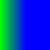`width = 25; height = 25; rotation = 0; tx = 25; ty = 0;`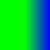`width = 50; height = 50; rotation = 0; tx = 0; ty = 0;`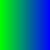`width = 50; height = 50; rotation = Math.PI / 4; // 45 degrees tx = 0; ty = 0;`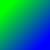Parameters:

`width` The width of the gradient box. The height of the gradient box. The amount to rotate, in radians. The distance, in pixels, to translate to the right along the x axis. This value is offset by half of the `width` parameter. The distance, in pixels, to translate down along the y axis. This value is offset by half of the `height` parameter.

### `deltaTransformPoint(point:Point):Point`

Given a point in the pretransform coordinate space, returns the coordinates of that point after the transformation occurs. Unlike the standard transformation applied using the `transformPoint()` method, the `deltaTransformPoint()` method's transformation does not consider the translation parameters `tx` and `ty`.

Parameters:

`point` The point for which you want to get the result of the matrix transformation.

Returns:

The point resulting from applying the matrix transformation.

### `identity():Void`

Sets each matrix property to a value that causes a null transformation. An object transformed by applying an identity matrix will be identical to the original. After calling the `identity()` method, the resulting matrix has the following properties: `a`=1, `b`=0, `c`=0, `d`=1, `tx`=0, `ty`=0.

In matrix notation, the identity matrix looks like this:### `invert():Matrix`

Performs the opposite transformation of the original matrix. You can apply an inverted matrix to an object to undo the transformation performed when applying the original matrix.

### `rotate(theta:Float):Void`

Applies a rotation transformation to the Matrix object. The `rotate()` method alters the `a`, `b`, `c`, and `d` properties of the Matrix object. In matrix notation, this is the same as concatenating the current matrix with the following:Parameters:

`angle` The rotation angle in radians.

### `scale(sx:Float, sy:Float):Void`

Applies a scaling transformation to the matrix. The x axis is multiplied by `sx`, and the y axis it is multiplied by `sy`. The `scale()` method alters the `a` and `d` properties of the Matrix object. In matrix notation, this is the same as concatenating the current matrix with the following matrix:Parameters:

`sx` A multiplier used to scale the object along the x axis. A multiplier used to scale the object along the y axis.

### `setTo(a:Float, b:Float, c:Float, d:Float, tx:Float, ty:Float):Void`

Sets the members of Matrix to the specified values

Parameters:

`aa` the values to set the matrix to. tya

### `toString():String`

Returns a text value listing the properties of the Matrix object.

Returns:

A string containing the values of the properties of the Matrix object: `a`, `b`, `c`, `d`, `tx`, and `ty`.

### `transformPoint(pos:Point):Point`

Returns the result of applying the geometric transformation represented by the Matrix object to the specified point.

Parameters:

`point` The point for which you want to get the result of the Matrix transformation.

Returns:

The point resulting from applying the Matrix transformation.

### `translate(dx:Float, dy:Float):Void`

Translates the matrix along the x and y axes, as specified by the `dx` and `dy` parameters.

Parameters:

`dx` The amount of movement along the x axis to the right, in pixels. The amount of movement down along the y axis, in pixels.